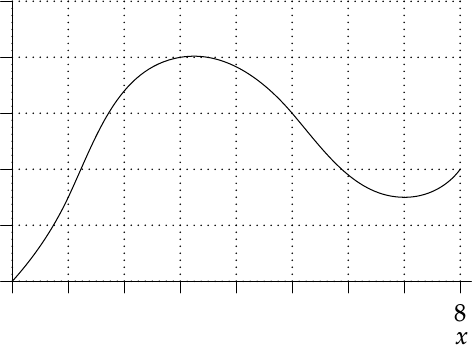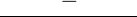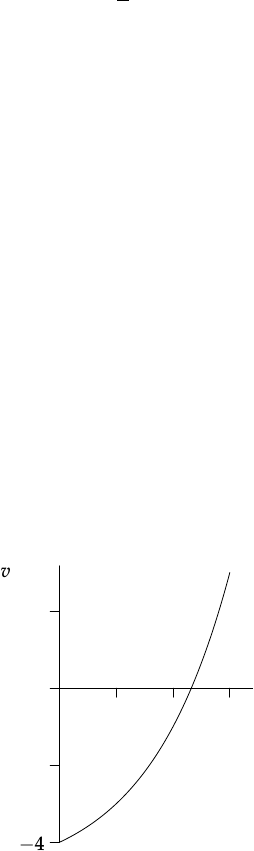Study Guides (390,000)
US (220,000)
UC-Irvine (3,000)
MATH (600)
MATH 2B (100)
All (60)
Final

# MATH 2B Study Guide - Final Guide: Riemann SumExam

Department
Mathematics
Course Code
MATH 2B
Professor
All
Study Guide
Final

This preview shows pages 1-3. to view the full 10 pages of the document.Math 2B: Sample Final 3
Turn off your cell phone and do not check it during the exam.
No calculators or other forms of assistance allowed.
This exam consists of 12 questions for 100 total points. Points per question are in brackets.
Read the directions for each problem carefully and answer all parts of each problem.
Unless instructed otherwise, show all work for full credit.
Deﬁne any notation used and label any sketches/graphs.
1. For the function drawn, estimate the area under the curve using a Riemann sum with four
subintervals and midpoints. Sketch the Riemann sum by drawing rectangles on the picture. (5)
0
1
2
3
4
5
f(x)
012345678
x

Only pages 1-3 are available for preview. Some parts have been intentionally blurred.2
2. Evaluate the following integrals
(a) Zsin θcos2θdθ(3)
(b) Zx7
(x+1)(x3)dx(8)

Only pages 1-3 are available for preview. Some parts have been intentionally blurred.3
3. Compute the average value fav of the function f(x) = xcos xon the interval [0, π
2].(7)
4. A particle has velocity v(t) = 2t5 ft/s at time tseconds.
(a) Compute the displacement of the particle over the time inter-
val t=0 to t=3. (4)
4
2
0
2
v
123
t
(b) The distance travelled by the particle over the same time interval is given by
Za
052tdt+Z3
a2t5dt
where ais a constant. What is the value of a?(2)